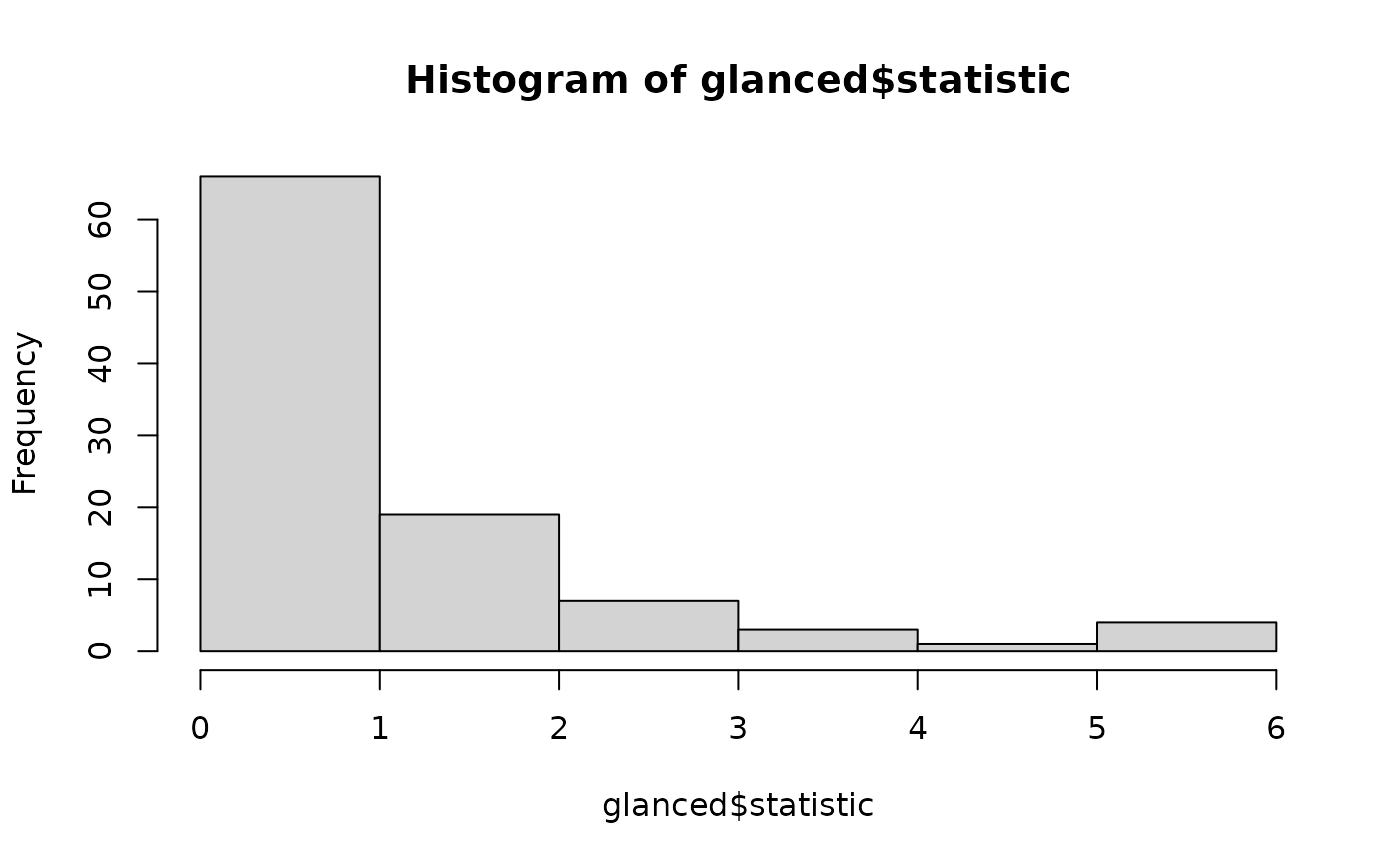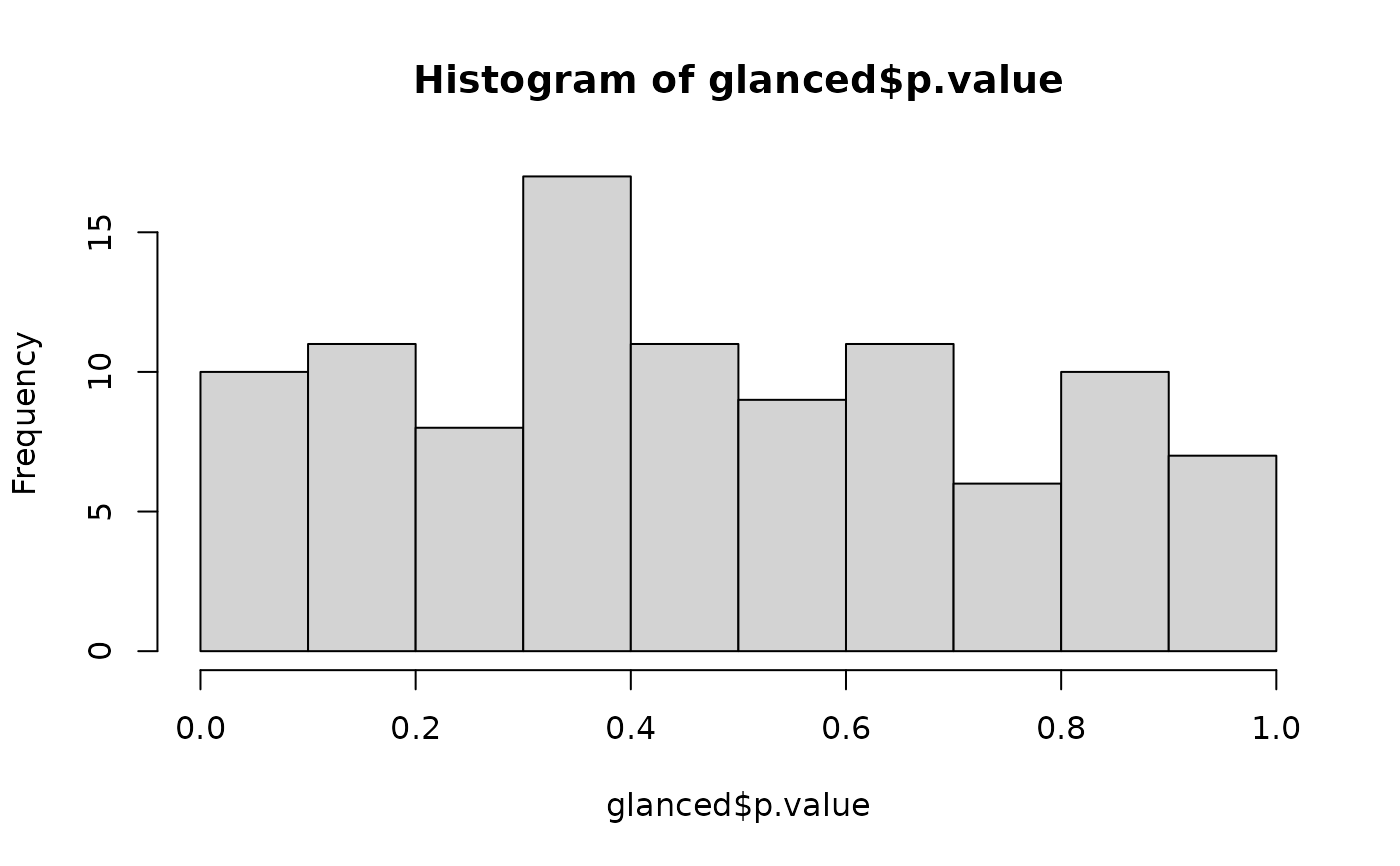A permutation test involves permuting one or more variables in a data set before performing the test, in order to break any existing relationships and simulate the null hypothesis. One can then compare the true statistic to the generated distribution of null statistics.

## Usage

permute(data, n, ..., .id = ".id")

permute_(data, n, columns, .id = ".id")

## Arguments

data

A data frame

n

Number of permutations to generate.

...

Columns to permute. This supports bare column names or dplyr dplyr::select_helpers

.id

Name of variable that gives each model a unique integer id.

columns

In permute_, vector of column names to permute.

## Value

A data frame with n rows and one column: perm

## Examples


library(purrr)
perms <- permute(mtcars, 100, mpg)

models <- map(perms$perm, ~ lm(mpg ~ wt, data = .)) glanced <- map_df(models, broom::glance, .id = "id") # distribution of null permutation statistics hist(glanced$statistic)# confirm these are roughly uniform p-values
hist(glanced$p.value)# test against the unpermuted model to get a permutation p-value mod <- lm(mpg ~ wt, mtcars) mean(glanced$statistic > broom::glance(mod)\$statistic)
#>  0